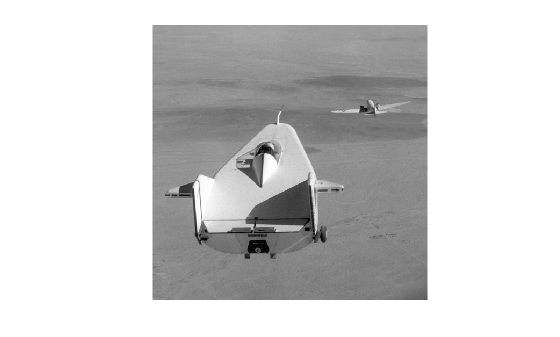# improfile

Pixel-value cross-sections along line segments

## Syntax

``c = improfile``
``c = improfile(n)``
``c = improfile(I,xi,yi)``
``c = improfile(xref,yref,I,xi,yi)``
``c = improfile(___,n)``
``c = improfile(___,method)``
``[cx,cy,c] = improfile(___)``
``[cx,cy,c,xi2,yi2] = improfile(___)``
``improfile(___)``

## Description

### Select Line Segments Interactively

````c = improfile` lets you select line segments interactively from the image in the current axes. When you finish selecting line segments, `improfile` returns sampled pixel values along the line segments in `c`.With this syntax, you specify the line or path using the mouse, by clicking points in the image. Press Backspace or Delete to remove the previously selected point. To finish selecting points, adding a final point, press shift-click, right-click, or double-click. To finish selecting points without adding a final point, press Return.```
````c = improfile(n)` returns `n` sampled pixel values from line segments that you select interactively.```

### Select Line Segments by Specifying Endpoints

````c = improfile(I,xi,yi)` returns sampled pixel values along line segments in image `I`. The endpoints of the line segments have (x, y) coordinates `xi` and `yi`.```
````c = improfile(xref,yref,I,xi,yi)` returns pixel values in the world coordinate system defined by `xref` and `yref`. The line segment endpoints have (x, y) coordinates `xi` and `yi` in this coordinate system.```
````c = improfile(___,n)` returns `n` sampled pixel values along the line segments.```

### Specify Interpolation Method or Output Options

````c = improfile(___,method)` specifies the interpolation method for pixel coordinates. Before the `method` input argument, you can specify the input arguments of any other syntax.```
````[cx,cy,c] = improfile(___)` additionally returns the (x, y) coordinates of the sampled pixels, `cx` and `cy`. You can use the input arguments of any other syntax.```
````[cx,cy,c,xi2,yi2] = improfile(___)` additionally returns the (x, y) coordinates of the line segment endpoints, `xi` and `yi`.```

example

````improfile(___)` without output arguments displays a plot of pixel values along the line segments. If you select a single line segment, then `improfile` creates a two-dimensional plot of intensity values versus the distance along the line segment. If you select two or more line segments, then `improfile` creates a three-dimensional plot of the intensity values versus their x- and y-coordinates.```

## Examples

collapse all

Read an image into the workspace, and display it.

```I = imread('liftingbody.png'); imshow(I)```Specify x- and y-coordinates that define connected line segments.

```x = [19 427 416 77]; y = [96 462 37 33];```

Display a 3-D plot of the pixel values of these line segments.

`improfile(I,x,y),grid on;`## Input Arguments

collapse all

Number of points to along the path to sample, specified as a positive integer. If you do not provide this argument, then `improfile` chooses a value for `n` that is roughly equal to the number of pixels that the path traverses.

Data Types: `double`

Input image, specified as an RGB image, grayscale image, or binary image.

Data Types: `single` | `double` | `int16` | `uint8` | `uint16` | `logical`

x-coordinate of line segment endpoints, specified as a numeric vector of the same length as `yi`. If you specify image limits in a world coordinate system using `xref`, then `xi` is in this coordinate system. Otherwise, `xi` is in the default spatial coordinate system.

Data Types: `double`

y-coordinate of line segment endpoints, specified as a numeric vector of the same length as `xi`. If you specify image limits in a world coordinate system using `yref`, then `yi` is in this coordinate system. Otherwise, `yi` is in the default spatial coordinate system.

Data Types: `double`

Image limits in world coordinates along the x-dimension, specified as a 2-element numeric vector of the form [xmin xmax]. The value of `xref` sets the image `XData`. The data type of `xref` and `yref` must match.

Data Types: `single` | `double`

Image limits in world coordinates along the y-dimension, specified as a 2-element numeric vector of the form [ymin ymax]. The value of `yref` sets the image `YData`. The data type of `xref` and `yref` must match.

Data Types: `single` | `double`

Interpolation method, specified as `'nearest'` for nearest-neighbor interpolation, `'bilinear'`, or `'bicubic'`.

Data Types: `char` | `string`

## Output Arguments

collapse all

Sampled pixel values, returned as an `n`-by-1 numeric vector when `I` is a grayscale or binary image, or an `n`-by-1-by-3 numeric array when `I` is an RGB image.

Data Types: `double`

x-coordinate of sampled pixels, returned as an `n`-by-1 numeric vector.

Data Types: `double`

y-coordinate of sampled pixels, returned as an `n`-by-1 numeric vector.

Data Types: `double`

x-coordinate of line segment endpoints, returned as a numeric vector. If you specify line segment endpoints using `xi`, then `xi2` is equal to `xi`.

Data Types: `double`

y-coordinate of line segment endpoints, returned as a numeric vector. If you specify line segment endpoints using `yi`, then `yi2` is equal to `yi`.

Data Types: `double`

## Version History

Introduced before R2006a